# Electronics and Communication Engineering - Analog Electronics

31.

A full wave rectifier circuit using centre tapped transformer, the input frequency 50 Hz. The frequency of output is

 A. 100 Hz B. 50 Hz C. 25 Hz D. none of the above

Explanation:

There are two output waves in one cycle of input wave.

32.

If the midband gain of an amplifier is 40 dB, the gain at half power frequency is

 A. 37 dB B. 30 dB C. 20 dB D. 13 dB

Explanation:

Half power frequency is -3 dB frequency.

33.

It is desired to reduce distortion in an amplifier from 8% to 2% by using 5% negative feedback. The open and closed loop gains respectively are

 A. 60 and 15 B. 15 and 60 C. 6 and 1.5 D. 1.5 and 6

Explanation: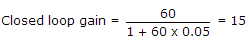.

34.

An ideal op-amp requires

 A. zero input offset voltage B. low input offset voltage C. high input offset voltage D. infinite input offset voltage

Explanation:

An ideal Op-amp has identical transistors and hence does not require input offset voltage.

35.

The JFET in the circuit shown in figure has an IDSS = 10 mA and Vp = -5V. The value of the resistance Rs for a drain current IDS = 6.4 mA is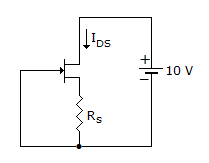A. 156 Ω B. 470 Ω C. 560 Ω D. 1 kΩ

Explanation: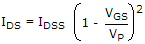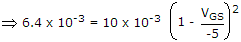VGS = - 1 VIDS.RS = - VGS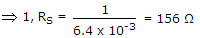.